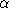## CGAL::Apollonius_graph_hierarchy_2<Gt,Agds>

### Definition

We provide an alternative to the class Apollonius_graph_2<Gt,Agds> for the dynamic construction of the Apollonius graph. The Apollonius_graph_hierarchy_2<Gt,Agds> class maintains a hierarchy of Apollonius graphs. The bottom-most level of the hierarchy contains the full Apollonius diagram. A site that is in level i, is in level i+1 with probability 1/where> 1 is some constant. The difference between the Apollonius_graph_2<Gt,Agds> class and the Apollonius_graph_hierarchy_2<Gt,Agds> is on how the nearest neighbor location is done. Given a point p the location is done as follows: at the top most level we find the nearest neighbor of p as in the Apollonius_graph_2<Gt,Agds> class. At every subsequent level i we use the nearest neighbor found at level i+1 to find the nearest neighbor at level i. This is a variant of the corresponding hierarchy for points found in [Dev98]. The class has two template parameters which have essentially the same meaning as in the Apollonius_graph_2<Gt,Agds> class. The first template parameter must be a model of the ApolloniusGraphTraits_2 concept. The second template parameter must be a model of the ApolloniusGraphDataStructure_2 concept. However, the vertex base class that is to be used in the Apollonius graph data structure must be a model of the ApolloniusGraphHierarchyVertexBase_2 concept. The second template parameter defaults to Triangulation_data_structure_2< Apollonius_graph_hierarchy_vertex_base_2< Apollonius_graph_vertex_base_2<Gt,true> >, Triangulation_face_base_2<Gt> >.

The Apollonius_graph_hierarchy_2<Gt,Agds> class derives publicly from the Apollonius_graph_2<Gt,Agds> class. The interface is the same with its base class. In the sequel only the methods overridden are documented.

#include <CGAL/Apollonius_graph_hierarchy_2.h>

### Inherits From

CGAL::Apollonius_graph_2<Gt,Agds>

### Types

Apollonius_graph_hierarchy_2<Gt,Agds> does not introduce other types than those introduced by its base class Apollonius_graph_2<Gt,Agds>.

### Creation

 Apollonius_graph_hierarchy_2 agh ( Gt gt=Gt()); Creates an hierarchy of Apollonius graphs using gt as geometric traits. template< class Input_iterator > Apollonius_graph_hierarchy_2 agh ( Input_iterator first, Input_iterator beyond, Gt gt=Gt()); Creates an Apollonius graph hierarchy using gt as geometric traits and inserts all sites in the range [first, beyond). Apollonius_graph_hierarchy_2 agh ( other); Copy constructor. All faces, vertices and inter-level pointers are duplicated. After the construction, agh and other refer to two different Apollonius graph hierarchies: if other is modified, agh is not.
 Apollonius_graph_hierarchy_2 agh = other Assignment. All faces, vertices and inter-level pointers are duplicated. After the construction, agh and other refer to two different Apollonius graph hierarchies: if other is modified, agh is not.

### Insertion

template< class Input_iterator >
unsigned int agh.insert ( Input_iterator first, Input_iterator beyond)
Inserts the sites in the range [first,beyond). The number of sites in the range [first, beyond) is returned.
 Precondition: Input_iterator must be a model of InputIterator and its value type must be Site_2.
Vertex_handle agh.insert ( Site_2 s) Inserts the site s in the Apollonius graph hierarchy. If s is visible then the vertex handle of s is returned, otherwise Vertex_handle(NULL) is returned.
Vertex_handle agh.insert ( Site_2 s, Vertex_handle vnear)
Inserts s in the Apollonius graph hierarchy using the site associated with vnear as an estimate for the nearest neighbor of the center of s. If s is visible then the vertex handle of s is returned, otherwise Vertex_handle(NULL) is returned. A call to this method is equivalent to agh.insert(s); and it has been added for the sake of conformity with the interface of the Apollonius_graph_2<Gt,Agds> class.

### Removal

void agh.remove ( Vertex_handle v) Removes the site associated to the vertex handle v from the Apollonius graph hierarchy.
 Precondition: v must correspond to a valid finite vertex of the Apollonius graph hierarchy.

### Nearest neighbor location

 Vertex_handle agh.nearest_neighbor ( Point p) Finds the nearest neighbor of the point p. In other words it finds the site whose Apollonius cell contains p. Ties are broken arbitrarily and one of the nearest neighbors of p is returned. If there are no visible sites in the Apollonius diagram Vertex_handle(NULL) is returned. Vertex_handle agh.nearest_neighbor ( Point p, Vertex_handle vnear) Finds the nearest neighbor of the point p. If there are no visible sites in the Apollonius diagram Vertex_handle(NULL) is returned. A call to this method is equivalent to agh.nearest_neighbor(p); and it has been added for the sake of conformity with the interface of the Apollonius_graph_2 class.

### I/O

 void agh.file_output ( std::ostream& os) Writes the current state of the Apollonius graph hierarchy to an output stream. In particular, all visible and hidden sites are written as well as the underlying combinatorial hierarchical data structure. void agh.file_input ( std::istream& is) Reads the state of the Apollonius graph hierarchy from an input stream. std::ostream& std::ostream& os << agh Writes the current state of the Apollonius graph hierarchy to an output stream. std::istream& std::istream& is >> agh Reads the state of the Apollonius graph hierarchy from an input stream.

### Validity check

 bool agh.is_valid ( bool verbose = false, int level = 1) Checks the validity of the Apollonius graph hierarchy. If verbose is true a short message is sent to std::cerr. If level is 0, the data structure at all levels is validated, as well as the inter-level pointers. If level is 1, then the data structure at all levels is validated, the inter-level pointers are validated and all levels of the Apollonius graph hierarchy are also validated. Negative values of level always return true, and values greater then 1 are equivalent to level being 1.

### Miscellaneous

 void agh.clear () Clears all contents of the Apollonius graph hierarchy. void agh.swap ( other) The Apollonius graph hierarchies other and agh are swapped. agh.swap(other) should be preferred to agh = other or to agh(other) if other is deleted afterwards.